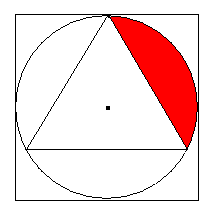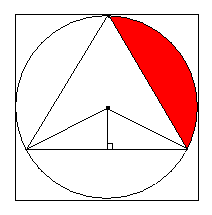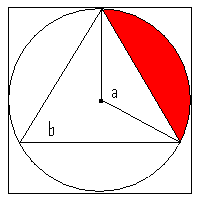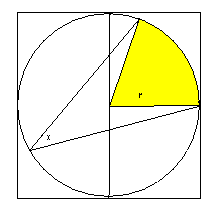# Geometry Series Part 2: Inscribed Triangles:

by on November 17th, 2009

To start off, let’s quickly review the essentials. These are formulas/concepts you must know:

1. a² + b² = c², but only when a right triangle. If you don’t know it’s a right triangle, Pythagorean theorem does not apply!
2. Common special right triangles include 3-4-5, 5-12-13, 8-15-17, 7-24-25 (and their multiples.)
3. 45-45-90 triangles are ALWAYS in the ratio 1:1:√2
4. 30-60-90 triangle are ALWAYS in the ratio 1:√3:2
5. Angles and opposite sides are in the same relative size order, but are NOT proportional.

Let’s continue with a standard diagram in which we have an equilateral triangle inscribed in a circle, which is inscribed in a square.The center point of all three figures (triangle, circle, square) are all the same, but this is ONLY true if the triangle is equilateral. Therefore, if given ANY piece of information about the circle, square or triangle, we can derive the rest. We draw a perpendicular line from the center to the side of the triangle.Note that the hypotenuses of the smaller triangles are equal to the radius of the circle. We also know that the smaller triangles are each 30-60-90 because you are taking the 120-degree internal angle from the circle’s center and cutting it in two. Here are your basic conversions:

r = ½d = ½s, where s is the side of the square.
The sides of the 30-60-90 triangles become ½r : (r√3)/2 : r respectively
The side of the equilateral triangle becomes 2*(r√3)/2 = r√3

If given the area of the square, we should be able to derive essentially any other information.

## Area of an Equilateral Triangle

The area of an equilateral triangle equals (s²√3)/4. Memorize this. It will save you the time of drawing a 30-60-90 triangle, solving for the base, finding the height, multiplying and dividing by 2. That was long to write, imagine how long it takes to do!

If  the area of the square = 64 and we needed to find the area of the triangle, we just use the conversions above:

d = 8
r = 4
side of triangle = 4√3

Area of triangle = [(4√3)²√3]/4 = 16*3*√3 / 4 = 4*3*√3 = 12√3

## Angle RelationshipsAnother important rule is that the interior angle created from of two radii extending to the outside of the circle is exactly twice the measure of any angle on the circle extending to those same points.  In the image above, 2b = a. This information is never explicitly stated on tests, but will come up on DS questions over and over.

Let’s take a look at this practice question:In the figure above, a circle is inscribed in a square. What is the area of the shaded region?

(1) The perimeter of the square equals 32.
(2) x = 35

Statement 1 seems irrelevant to the question, but we can determine r by knowing that the length of the square’s side. If s = 32/4 = 8, then d = 8 and r = 4. This is insufficient, since we do not know the interior angle.

Statement 2 provides information about x, and from this, we know that the interior angle of the shaded region is 2(35) = 70. This is insufficient, since we do not know the size of the circle.

Together, we know both the size of the circle and the degree measure of the interior sector angle.

A(shaded) = (2x/360) * πr² = 70/360 * 16π, whatever the hell that comes out to. Remember, since it’s a Data Sufficiency question, we don’t actually need to calculate the number.

There are infinite variations of these concepts. Be flexible in your reasoning, and practice makes perfect!

Good luck!

•"We also know that the smaller triangles are each 30-60-90 because you are taking the 120-degree internal angle from the circle’s center and cutting it in two"

How do we know that the angle we make is 120?

•The large triangle is equilateral, so the 3 internal angles created from its center extending to its vertices are all 120. Another way to think about it is that the internal angles of all equi triangles are 60, so we can use the 1/2 angle rule above.

•"We also know that the smaller triangles are each 30-60-90 because you are taking the 120-degree internal angle from the circle’s center and cutting it in two."

How do we know that this angle is 120?

•Let me put it this way:
Sum of all angles of a triangle: 180 degrees
Sides opposite to equal angles are equal
Since its an equilateral triangle all the sides are equal. Therefore, each angle measure is 60 degrees.
Now, Angle subtended by an arc at the center of a circle is twice the measure of angle subtended anywhere else.
Therefore,
2x60=120
Hope this helps.

•The above statement i.e 120 degrees internal triangle holds true only in case of equilateral triangle .How about other triangles??

•Itreally a great and helpful piece of information. I am happy that you shared this useful information with us. Please keep us up to date like this. Thank you for sharing.